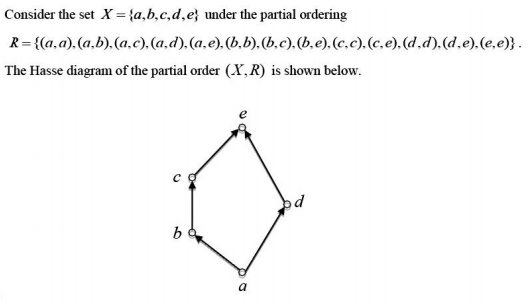# GATE | GATE-CS-2017 (Set 2) | Question 33The minimum number of ordered pairs that need to be added to R to make (X, R) a lattice is _____.

Note: This question appeared as Numerical Answer Type.
(A) 0
(B) 1
(C) 2
(D) 3

Explanation:

```Here in the given lattice a should be related as
(a,a) , (a,b), (a,c), (a,d), (a,e)

b: (b,c), (b,e), (b,b).

c: (c,c), (c,e)

d: (d,d), (d,e)

e: (e,e)

all pairs are already present here .